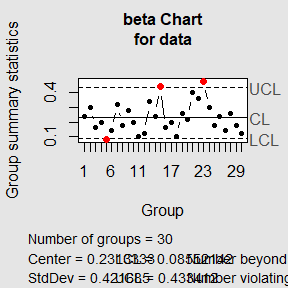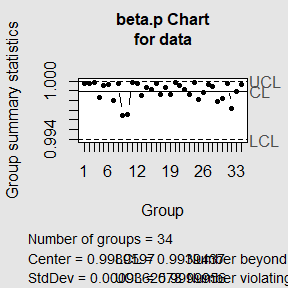library(bcc)

Introduction

qcc is a package for statistical process control and control charts built based on the qcc package, with new functions allowing the use of beta control charts.

This package allows the user to:

• build beta control charts

As the package uses the qcc package as the basis for building the graphics, all qcc functions and utilities also work on bcc. For more informations please consider visiting https://luca-scr.github.io/qcc/.

Application

You can use bcc in two basic ways:

• discrete data representing the sample size using type = 1;

• continuous data without sample size with values in the range between [0.1] using type = 2; you can make a ratio between the data to be analyzed for example.

Beta Charts

Beta Chart for discrete data

data("Montgomery2005")
bcc(data=Montgomery2005$Defective, sizes = Montgomery2005$Sample, type=1)Beta chart for continuos data

data("Drapper1998data")
bcc(data = Drapper1998data, type = "2")#> List of 11
#>  $call : language qcc(data = data, type = "beta.p", center = center, std.dev = std.dev, limits = limits, data.name = data.name| __truncated__ ... #>$ type            : chr "beta.p"
#>  $data.name : chr "data" #>$ data            : num [1:34, 1] 1 1 1 0.998 1 ...
#>   ..- attr(*, "dimnames")=List of 2
#>  $statistics : Named num [1:34] 1 1 1 0.998 1 ... #> ..- attr(*, "names")= chr [1:34] "1" "2" "3" "4" ... #>$ sizes           : int [1:34] 1 1 1 1 1 1 1 1 1 1 ...
#>  $center : num 0.999 #>$ std.dev         : num 0.000936
#>  $confidence.level: num 0.9 #>$ limits          : num [1, 1:2] 0.994 1
#>   ..- attr(*, "dimnames")=List of 2
#>  \$ violations      :List of 2
#>  - attr(*, "class")= chr "qcc"